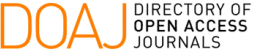### Numerical Study for Two-Phase Flow with Gravity in Homogeneous and Piecewise-Homogeneous Porous Media

Isamara L. N. Araujo, Panters Rodríguez-Bermúdez, Yoisell Rodríguez-Núñez

#### Abstract

In this work we study two-phase flow with gravity either in 1-rock homogeneous media or 2-rocks composed media, this phenomenon can be modeled by a non-linear scalar conservation law with continuous flux function or discontinuous flux function, respectively. Our study is essentially from a numerical point of view, we apply the new Lagrangian-Eulerian finite difference method developed by Abreu and Pérez  and the Lax-Friedrichs classic method to obtain numerical entropic solutions. Comparisons between numerical and analytical solutions show the efficiency of the methods even for discontinuous flux function.

#### Keywords

Conservation laws; finite differences methods; Lagrangian-Eulerian approach; two-phase flow; heterogeneous porous medium

PDF

#### References

J. A. Perez, Lagrangian-Eulerian approximation methods for balance laws and hyperbolic conservation laws. Ph.d. thesis, 2015.

G. B. Whitham, Linear and Nonlinear Waves. Wiley-Interscience, 1999.

C. Dafermos, Hyperbolic Conservation Laws in Continuum Physics. Springer, 2000.

P. D. Lax, “Hyperbolic systems of conservation laws ii,” Comm. Pure Appl. Math., vol. 10, pp. 537–566, 1957.

H. W. Liepmann and A. Roshko, Elements of gasdynamics. John Wiley & Sons, 1959.

C. J. van Duijn, An Introduction to Conservations Laws: Theory and Applications to Multi-Phase Flow. Lecture Notes, Delft University of Technology, 2002.

S. Buckley and M. Leverett, “Mechanims of fluid displacement in sands,” Trans. AIME, no. 146, pp. 187–196, 1942.

W. Proskurowski, “A note on solving the buckley-leverett equation in the presence of gravity,” J. Comput. Phys., no. 41, pp. 136–141, 1981.

D. Marchesin, E. Isaacson, B. Plohr, and J. B. Temple, “Multiphase flow models with singular riemann problems,” Computation and Applied Mathematics, vol. 11, no. 2, pp. 147–166, 1992.

P. Castaneda, E. Abreu, F. Furtado, and D. Marchesin, “On a universal structure for immiscible three-phase flow in virgin reservoirs.,” Computational Geosciences, vol. 20, pp. 171–185, 2016.

P. Rodríguez-Bermúdez and D. Marchesin, “Riemann solutions for vertical flow of three phases in porous media: simple cases,” Journal of Hyperbolic Differential Equations, 2013.

R. J. LeVeque, Numerical Methods for Conservation Laws. Basel; Boston; Berlin: Birkhauser, second ed., 1992.

O. A. Oleinik, “On the uniqueness of generalized solution of cauchy problem for non linear system of equations occurring in mechanics,” Uspekhi Mat. Nauk, no. 12, pp. 3–73, 1957.

R. J. LeVeque, Numerical Methods for Conservation Laws. Birkhauser, second ed., 1992.

A. Kantzas, J. Bryan, and S. Taheri, “Fundamentals of

fluid flow in porous media.” http://perminc.com/resources/

fundamentals-of-fluid-flow-in-porous-media/, S.I. Acesso em 31

de agosto de 2018.

A. Riaz and H. Tchelepi, “Dynamics of vertical displacement in porous media associated with co2 sequestration,” SPE Journal, 2008.

A. Riaz and H. A. Tchelepi, “Stability of two-phase vertical flow in homogeneous porous media,” Phys. Fluids, vol. 19, July 2007. DOI:10.1063/1.2742975.

M. Wangen, “Vertical migration of hydrocarbons modeled with fractional flow theory,” Geophysical Journal International, vol. 115, pp. 109–131, 1993.

E. Kaasschieter, “Solving the buckley leverett equation with gravity in a heterogeneous porous medium,” Comp. Geosci., vol. 3, 1999.

M. Hayek, E. Mouche, and C. Mugler, “Modeling vertical stratification of co2 injected into deeep layered aquifier,” in Advances in Water Resours, vol. 32, pp. 450–462, 2009.

B. Andreianov and C. Cancès, “Vanishing capillarity solutions of buckleyleverett equation with gravity in two-rocks medium,” Comput. Geosci., vol. 17,

no. 3, pp. 551–572, 2013.

E. C. Abreu and J. A. Perez, “A lagrangian-eulerian algorithm scheme for hyperbolic conservation laws and balance laws.,” HYP2014- XV International Conference on Hyperbolic Problems, 2014. Acesso em 31 de agosto de 2018.

E. C. Abreu and J. A. Perez, “A new locally conservative lagrangian eulerian method for hyperbolic and balance laws.,” VIII Pan-American Workshop Applied and Computational Mathematics,, 2014.

O. Oleinik, “Discontinuous solutions of nonlinear differential equations,” Amer. Math. Soc. Trans. Ser., vol. 26, pp. 95–172, 1957.

D. Kröner, Numerical Schemes for Conservation Laws. Stuttgart: John Wiley Sons, 1997.

R. J. LeVeque, Finite Difference Methods for Ordinary and Partial Differential Equations. SIAM, 2007.

DOI: https://doi.org/10.5540/tema.2020.021.01.21

### Refbacks

• There are currently no refbacks.

Trends in Computational and Applied Mathematics

A publication of the Brazilian Society of Applied and Computational Mathematics (SBMAC)

Indexed in: